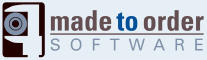# Less Than (typed)

SWF Action
Action Category:
Comparisons
Action Details:
(typed)
Action Identifier:
72
Action Structure:
<n.a.>
Action Length:
0 byte(s)
Action Stack:
pop 2 (a), push 1 (b)
Action Operation:
a1 := pop();
a2 := pop();
if(is_int(a1) && is_int(a2)) {
i1 := (int)a1;
i2 := (int)a2;
r := i2 < i1;    // compare integers
}
else if(is_numeric(a1) && is_numeric(a2)) {
f1 := (float)a1;
f2 := (float)a2;
r := f2 < f1;    // compare floating points
}
else {
s1 := (string)a1;
s2 := (string)a2;
r := s2 < s1;    // compare characters, case sensitive
}
push(r);
Action Flash Version:
5